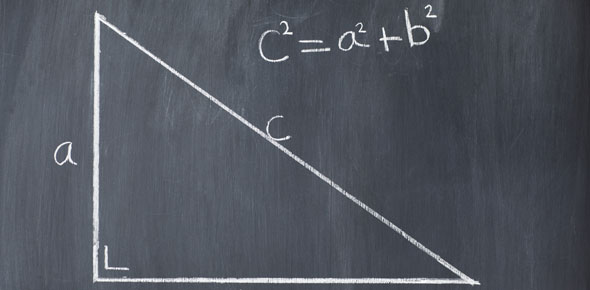# Right Triangle Worksheet

5 Questions | Total Attempts: 31SettingsUsing the Pythagorean Theorem, complete the following right triangle problems.

Related Topics
• 1.
In the diagram, what is a?
• A.

5.7m

• B.

1.3m

• C.

0.3m

• D.

4.0m

• 2.
Find the length of the missing leg.
• A.

28.3

• B.

11

• C.

73

• D.

52

• 3.
In the following diagram what is a? If c=25, and p=9
• A.

16

• B.

12

• C.

15

• D.

18

• 4.
Do the following sides make a right triangle? a=45, b=60, and c=75
• A.

True

• B.

False

• 5.
What is the length of the missing leg b, if a=8, and c=10?
• A.

3

• B.

12

• C.

18

• D.

6